Examples

Chapter 4 Class 11 Mathematical Induction
Serial order wise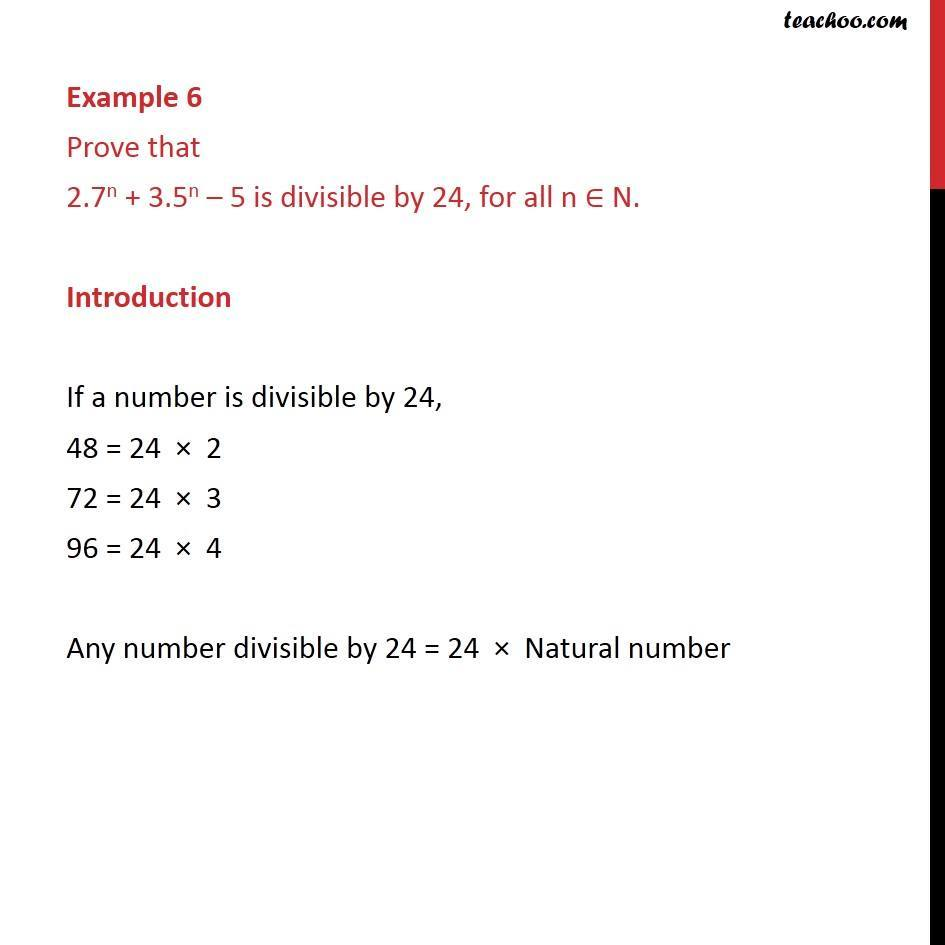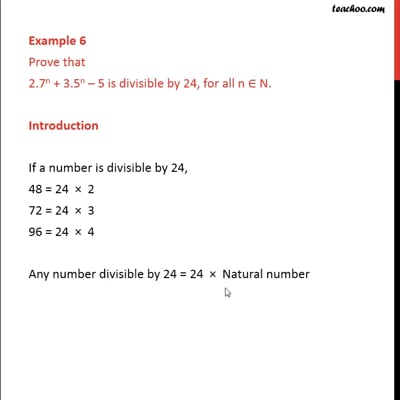This video is only available for Teachoo black users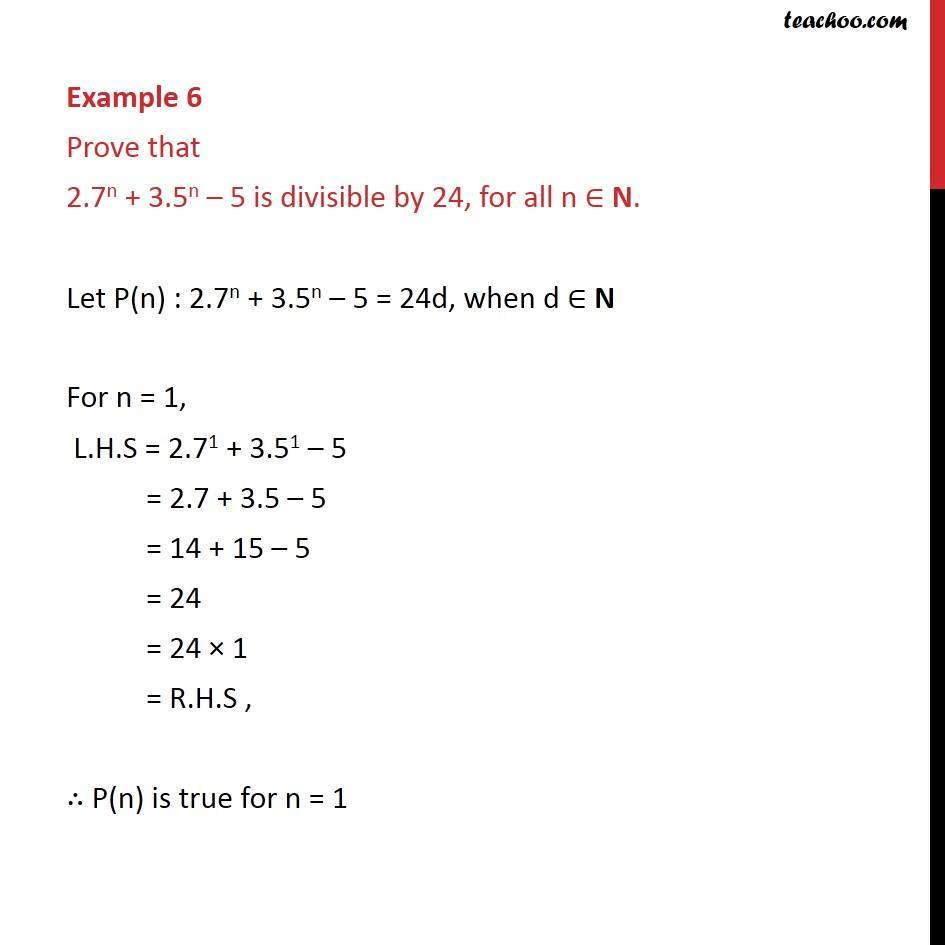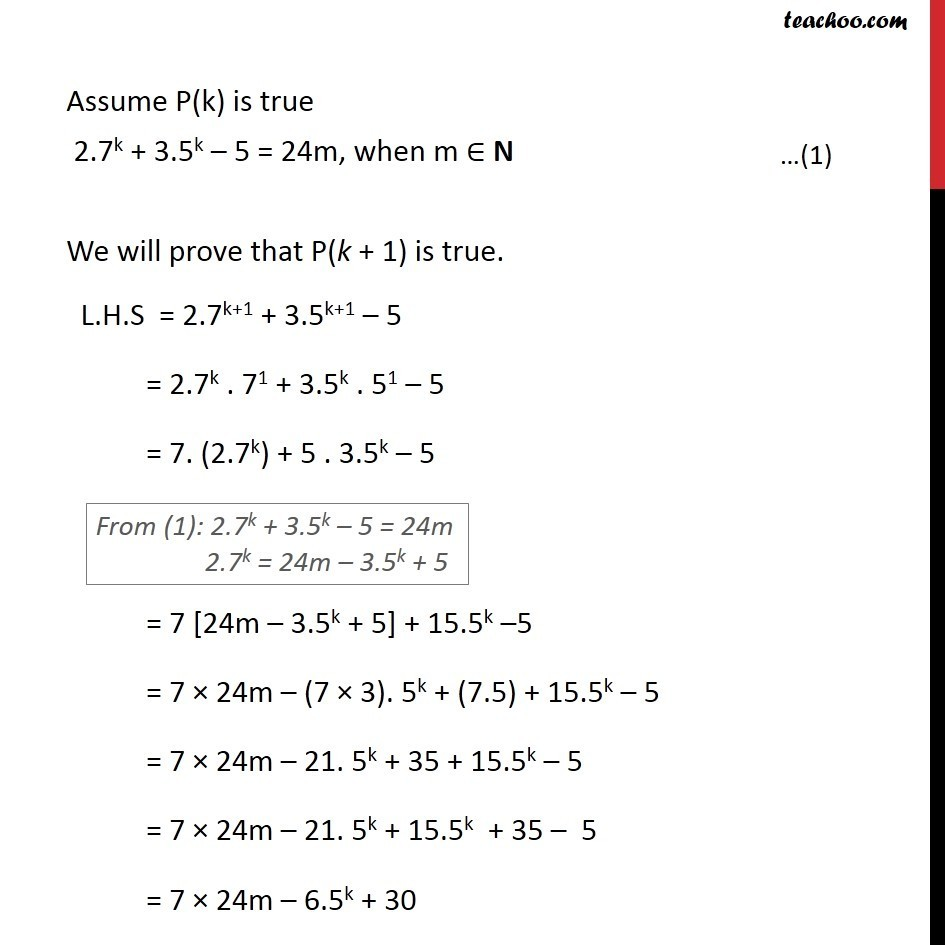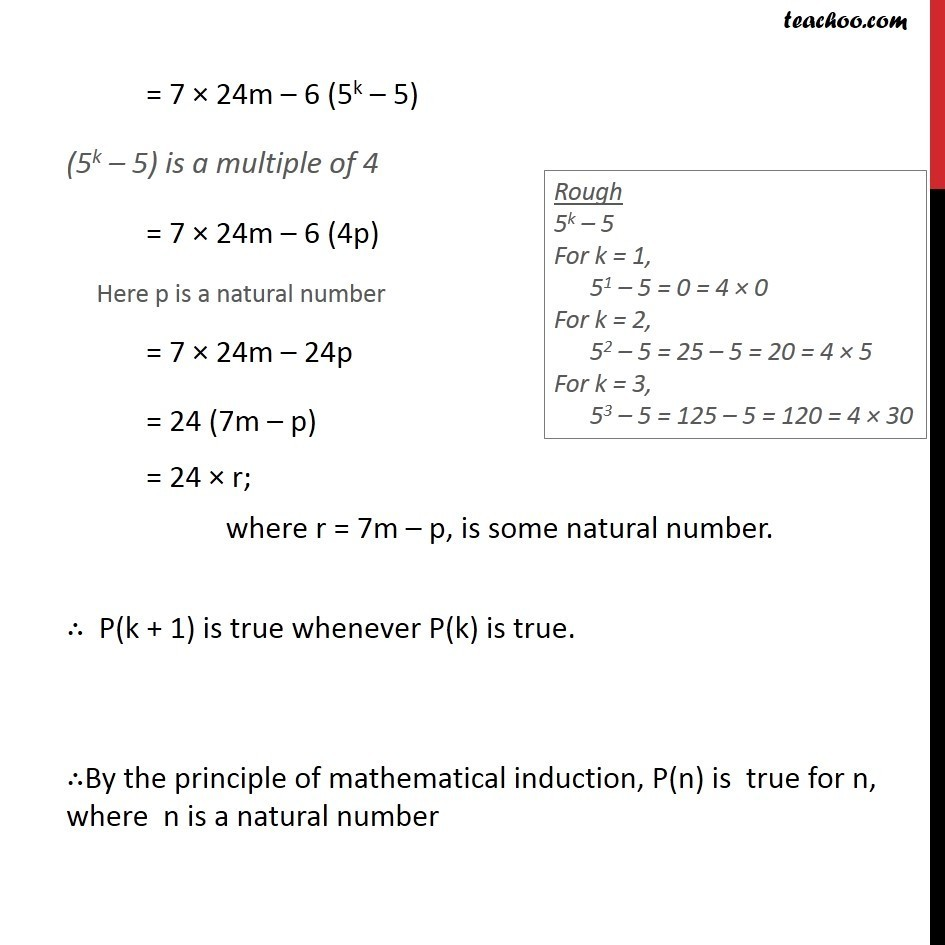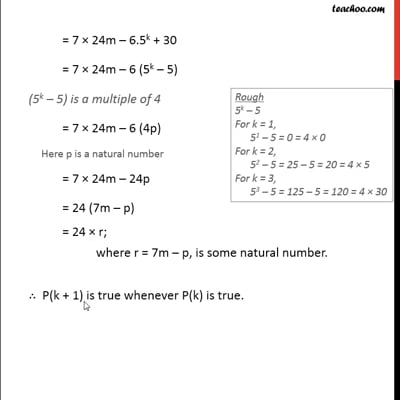This video is only available for Teachoo black users

Solve all your doubts with Teachoo Black (new monthly pack available now!)

### Transcript

Example 6 Prove that 2.7n + 3.5n 5 is divisible by 24, for all n N. Introduction If a number is divisible by 24, 48 = 24 2 72 = 24 3 96 = 24 4 Any number divisible by 24 = 24 Natural number Example 6 Prove that 2.7n + 3.5n 5 is divisible by 24, for all n N. Let P(n) : 2.7n + 3.5n 5 = 24d, when d N For n = 1, L.H.S = 2.71 + 3.51 5 = 2.7 + 3.5 5 = 14 + 15 5 = 24 = 24 1 = R.H.S , P(n) is true for n = 1 Assume P(k) is true 2.7k + 3.5k 5 = 24m, when m N We will prove that P(k + 1) is true. L.H.S = 2.7k+1 + 3.5k+1 5 = 2.7k . 71 + 3.5k . 51 5 = 7. (2.7k) + 5 . 3.5k 5 = 7 [24m 3.5k + 5] + 15.5k 5 = 7 24m (7 3). 5k + (7.5) + 15.5k 5 = 7 24m 21. 5k + 35 + 15.5k 5 = 7 24m 21. 5k + 15.5k + 35 5 = 7 24m 6.5k + 30 = 7 24m 6 (5k 5) (5k 5) is a multiple of 4 = 7 24m 6 (4p) = 7 24m 24p = 24 (7m p) = 24 r; where r = 7m p, is some natural number. P(k + 1) is true whenever P(k) is true. By the principle of mathematical induction, P(n) is true for n, where n is a natural number